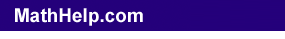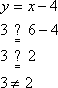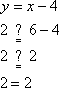Search

Systems of Non-Linear Equations:
Definitions
(page 1 of 6)A "system" of equations is a set of equations that you have to deal with all together. That is, you're dealing with more than one equation at once when you're dealing with a system of equations.

Think back to when you were first learning about equations. "Solutions" to equations were the points that made the equation true, that made the equation work correctly. For instance, in the equation "2x = 6", the "solution" is "x = 3" because, if you plug in 3 for x, you will get a true statement:  2(3) = 6 = 6. On the other hand, "x = 3" is not a solution to the equation, because plugging 3 in for x would create a false statement:  2×3 = 6 < > 6. (Note: "< >" is Internet shorthand for "does not equal" or "is not equal to".)

Eventually, you moved on to two-variable equations, such as "y = 3x + 2". You could "evaluate" these equations (usually meaning that you were given a value for x which you plugged in, and then you solved for the value of y), or you could graph them. You could also check solutions. For instance, suppose you were given "y = x  4". Is the point (6, 3) a solution? No, because plugging in 6 for x and 3 for y makes the equation false: Copyright © 2002-2011 Elizabeth Stapel All Rights ReservedSo (6, 3) is not a solution to this equation. But (6, 2) is:You would not usually work with the equation in this way, of course; you would usually be picking x-values, plugging them into the equation, finding the corresponding y-values, plotting the points, and graphing the line. For instance, you would pick 6 for x, plug this in to the equation, and compute y as:

y = x  4
y = 6  4
y = 2

However, the result is the same: you would have shown, in either case, that (6, 2) is a solution to the equation. That's the important thing to see here:

"SOLUTIONS" FOR EQUATIONS
ARE
"POINTS" ON THE GRAPHS

When you are solving a system of equations, you are looking for the points that are solutions for all of the system's equations. In other words, you are looking for the points that are solutions for all of the equations at once. What does this mean...?

Top  |  1 | 2 | 3 | 4 | 5 | 6  |  Return to Index  Next >>

 Cite this article as: Stapel, Elizabeth. "Systems of Non-Linear Equations: Definitions." Purplemath. Available from     https://www.purplemath.com/modules/syseqgen.htm. Accessed [Date] [Month] 2016